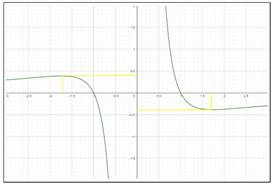# the local maximum and minimum values of the provided function.### Precalculus: Mathematics for Calcu...

6th Edition
Stewart + 5 others
Publisher: Cengage Learning
ISBN: 9780840068071### Precalculus: Mathematics for Calcu...

6th Edition
Stewart + 5 others
Publisher: Cengage Learning
ISBN: 9780840068071

#### Solutions

Chapter 3.1, Problem 61E
To determine

## the local maximum and minimum values of the provided function.

Expert Solution

The local maximum is 0.4 and local minimum is 0.4 .

### Explanation of Solution

Given information: the function provided in the question is,

V(x)=1x2x3

From the provided information draw a graph using the graphing calculator,Local maximum value of f ( a ) on a graph of f is the highest value on an interval containing a . Therefore, the local maximum value on the provided graph of f is 0.4, at x = -1.7.

The local minimum value of f ( a ) on a graph of f is the lowest value on an interval containing a . The local minimum value on the provided graph is -0.4, at x=1.7

Hence, the local maximum is 0.4 and local minimum is 0.4 .

### Have a homework question?

Subscribe to bartleby learn! Ask subject matter experts 30 homework questions each month. Plus, you’ll have access to millions of step-by-step textbook answers!